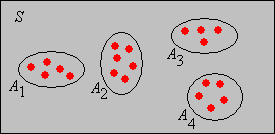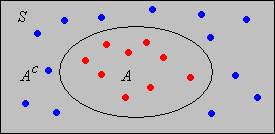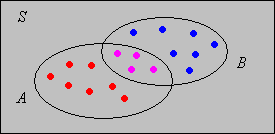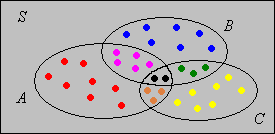]> Counting Measure

7. Counting Measure

Definitions and Basic Properties

Suppose that $S$ is a finite set. If $A S$ then the cardinality of $A$ is the number of elements in $A$, and is denoted $A$. The function  is called counting measure. Counting measure plays a fundamental role in discrete probability structures, and particularly those that involve sampling from a finite set. The set $S$ is typically very large, hence efficient counting methods are essential. The first combinatorial problem is attributed to the Greek mathematician Xenocrates.

In many cases, a set of objects can be counted by establishing a one-to-one correspondence between the given set and some other set. Naturally, the two sets have the same number of elements, but for some reason, the second set may be easier to count.

The addition rule of combinatorics is simply the additivity axiom of counting measure. If $A 1 A 2 A n$ is a collection of disjoint subsets of $S$ then

$i 1 n A i i 1 n A i$Simple Properties

The counting rules in this subsection are simple consequences of the addition rule.

Show that $A S A$.

Hint: $A$ and $A$ are disjoint and their union is $S$.Show that $B A B B A$.

Hint: $A B$ and $B A$ are disjoint and their union is $B$.

Show that if $A B$ then $B A B A$.

Hint: Apply the difference rule and note that $A B A$

Show that if $A B$ then $A B$.

Thus,  is an increasing function, relative to the subset partial order on $S$, and the ordinary order on .

Inequalities

This subsection gives two inequalities that are useful for obtaining bounds on the number of elements in a set.

Suppose that $A 1 A 2 A n$ is a a finite collection of subsets of $S$. Prove Boole's inequality (named after George Boole):

$i 1 n A i i 1 n A i$
1. Define $B 1 A 1$ and $B i A i A 1 A i 1$ for $i 2 3 n$.
2. Show that $B 1 B 2 B n$ are pairwise disjoint and have the same union as $A 1 A 2 A n$.
4. Use the increasing property.

Intuitively, Boole's inequality holds because parts of the union have been counted more than once in the expression on the right.

Suppose that $A 1 A 2 A n$ is a finite collection of subsets of $S$. Prove Bonferroni's inequality (named after Carlo Bonferroni):

$i 1 n A i S i 1 n S A i$

Hint: Apply Boole's inequality to $A 1 A 2 A n$ and use DeMorgan's law.

The Inclusion-Exclusion Formula

Show that $A B A B A B$

1. Note first that $A B A B A$ and the latter two sets are disjoint.
2. Now use the addition rule and the difference rule.Show that $A B C A + B + C − A B − A C − B C + A B C$

Hint: Use the inclusion-exclusion rule for two sets. You will use this rule three times.Exercise 7 and Exercise 8 can be generalized to a union of $n$ sets; the generalization is known as the inclusion-exclusion formula.

Suppose that $A i i I$ is a collection of subsets of $S$ where $I n$. Show that

$i I A i k 1 n 1 k 1 J I J k j J A j$

The general Bonferroni inequalities, named again for Carlo Bonferroni, state that if sum on the right is truncated after $k$ terms ($k n$), then the truncated sum is an upper bound for the cardinality of the union if $k$ is odd (so that the last term has a positive sign) and is a lower bound for the cardinality of the union if $k$ is even (so that the last terms has a negative sign).

The Multiplication Rule

The multiplication rule of combinatorics is based on the formulation of a procedure (or algorithm) that generates the objects to be counted. Specifically, suppose that the procedure consists of $k$ steps, performed sequentially, and that for each $j$, step $j$ can be performed in $n j$ ways regardless of the choices made on the previous steps. Then the number of ways to perform the entire algorithm (and hence the number of objects) is $n 1 n 2 n k$.

The key to a successful application of the multiplication rule to a counting problem is the clear formulation of an algorithm that generates the objects being counted, so that each object is generated once and only once. That is, we must neither over-count nor under-count.

The first two exercises below give equivalent formulations of the multiplication principle.

Suppose that $S$ is a set of sequences of length $k$, and that we denote a generic element of $S$ by $x 1 x 2 x k$. Suppose that for each $j$, $x j$ has $n j$ different values, regardless of the values of the previous coordinates. Show that

$S n 1 n 2 n k$

Suppose that $T$ is an ordered tree with depth $k$ and that each vertex at level $i 1$ has $n i$ children for $i 1 2 k$. Show that the number of vertices at level $k$ is $n 1 n 2 n k$.

Product Sets

Suppose that $S i$ is a set with $n i$ elements for $i 1 2 k$. Show that

$S 1 S 2 S k n 1 n 2 n k$

Show that if $S$ is a set with $n$ elements, then $S k$ has $n k$ elements.

Show that the number of ordered samples of size $k$ that can be chosen with replacement from a population of $n$ objects is $n k$.

Functions and Bit Strings

Show that the number of functions from a set $A$ of $n$ elements into a set $B$ of $m$ elements is $m n$.

Hint: To construct $f A B$, there are $m$ choices for $f x$ for each $x A$.

Recall that the set of functions from a set $A$ into a set $B$ (regardless of whether the sets are finite or infinite) is denoted $B A$. The result in the previous exercise is motivation for this notation.

Elements of $0 1 n$ are sometimes called bit strings of length $n$. Show that the number of such strings is $2 n$.

Subsets

Suppose that $S$ is a set with $n$ elements. Show that there are $2 n$ subsets of $S$.

Hint: To construct $A S$, decide whether $x A$ or $x A$ for each $x S$.

Suppose that $A 1 A 2 A k$ is a collection of $n$ subsets of a set $S$. Show that there are $2 2 k$ different (in general) sets that can be constructed from the $k$ given sets, using the operations of union, intersection, and complement. These sets form the algebra generated by the given sets.

1. Show that there are $2 k$ pairwise disjoint sets of the form $B 1 B 2 B k$ where $B i A i$ or $B i A i$ for each $i$.
2. Argue that every set that can be constructed from $A 1 A 2 A k$ is a union of some (perhaps all, perhaps none) of the sets in (a).

In the Venn diagram applet, observe the diagram of each of the 16 sets that can be constructed from $A$ and $B$.

Suppose that $S$ is a set with $n$ elements and that $A$ is a subset of $S$ with $k$ elements. Show that the number of subsets of $S$ that contain $A$ is $2 n k$.

Computational Exercises

A license number consists of two letters (uppercase) followed by five digits. How many different license numbers are there?

Suppose that a Personal Identification Number (PIN) is a four-symbol code word in which each entry is either a letter (uppercase) or a digit. How many PINs are there?

In the board game Clue, Mr. Boddy has been murdered. There are 6 suspects, 6 possible weapons, and 9 possible rooms for the murder.

1. The game includes a card for each suspect, each weapon, and each room. How many cards are there?
2. The outcome of the game is a sequence consisting of a suspect, a weapon, and a room (for example, Colonel Mustard with the knife in the billiard room). How many outcomes are there?
3. Once the three cards that constitute the outcome have been randomly chosen, the remaining cards are dealt to the players. Suppose that you are dealt 5 cards. In trying to guess the outcome, what hand of cards would be best?

An experiment consists of rolling a fair die, drawing a card from a standard deck, and tossing a fair coin. How many outcomes are there?

A fair die is rolled 5 times and the sequence of scores recorded. How many outcomes are there?

Suppose that 10 persons are chosen and their birthdays recorded. How many possible outcomes are there?

In the card game Set, each card has 4 properties: number (one, two, or three), shape (diamond, oval, or squiggle), color (red, blue, or green), and shading (solid, open, or stripped). The deck has one card of each (number, shape, color, shading) configuration. A set in the game is defined as a set of three cards which, for each property, the cards are either all the same or all different.

1. How many cards are in a deck?
2. How many sets are there?

A fair coin is tossed 10 times and the sequence of scores recorded.

1. How many sequences are there?
2. How many sequences are there that contain exactly 3 heads?

A string of lights has 20 bulbs, each of which may be good or defective. How many configurations are there?

The die-coin experiment consists of rolling a die and then tossing a coin the number of times shown on the die. The sequence of coin results is recorded.

1. How many outcomes are there?
2. How many outcomes are there with exactly 2 heads?

Run the die-coin experiment until exactly 2 heads occurs.

Suppose that a sandwich at a restaurant consists of bread, meat, cheese, and various toppings. There are 4 different types of bread (select one), 3 different types of meat (select one), 5 different types of cheese (select one), and 10 different toppings (select any). How many sandwiches are there?

The Galton Board

The Galton Board, named after Francis Galton, is a triangular array of pegs. Galton, apparently too modest to name the device after himself, called it a quincunx from the Latin word for five twelfths (go figure). The rows are numbered, from the top down, by $0 1 2$. Row $k$ has $k 1$ pegs that are labeled, from left to right by $0 1 k$. Thus, a peg can be uniquely identified by an ordered pair $i j$ where $i$ is the row number and $j$ is the peg number in that row.

A ball is dropped onto the top peg $0 0$ of the Galton board. In general, when the ball hits peg $i j$, it either bounces to the left to peg $i 1 j$ or to the right to peg $i 1 j 1$ The sequence of pegs that the ball hits is a path in the Galton board.

Show that there is a one-to-one correspondence between each pair of the following three collections:

1. Bit strings of length $n$
2. Paths in the Galton board from $0 0$ to a peg in row $n$.
3. Subsets of a set with $n$ elements.

Thus, from the previous exercise, each of these collections has $2 n$ elements.

Open the Galton board applet.

1. Move the ball from $0 0$ to $10 6$ along a path of your choice. Note the corresponding bit string and subset.
2. Generate the bit string $011100101$. Note the corresponding subset and path.
3. Generate the subset $2 4 5 9 12$. Note the corresponding bit string and path.
4. Generate all paths from $0 0$ to $4 2$. How many paths are there?